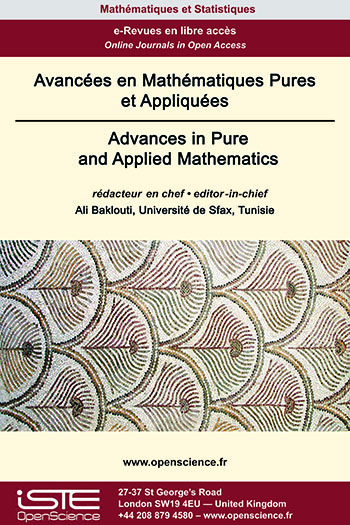# Vol 14 - Issue 4 (September 2023)

## List of Articles

Weyl almost automorphic functions and applications
M. Kostić

In this paper, we reconsider the notion of a Weyl p-almost automorphic function introduced by S. Abbas  in 2012 and propose several new ways for introduction of the class of Weyl p-almost automorphic functions (1 ⩽ p < ∞). We first analyze the introduced classes of Weyl p-almost automorphic functions of type 1, jointly Weyl p-almost automorphic functions and Weyl p-almost automorphic functions of type 2 in the one-dimensional setting. After that, we introduce and analyze generalizations of these classes in the multi-dimensional setting, working with general Lebesgue spaces with variable exponents. We provide several illustrative examples and applications to the abstract Volterra integro-differential equations.

Existence and multiplicity of solutions for α(x)-Kirchhoff Equation with indefinite weights
Khaled Ben Ali, Khaled Kefi, Haikel Ouerghi

In this paper, we investigate the existence of at least three weak solutions for a class of nonlocal elliptic equations with Navier boundary value conditions. The proof of our result uses the basic theory and critical point theory of variable exponential Lebesgue Sobolev spaces. Moreover a generalization of Corollary 1.1 in  is obtained.

Some remarks on surjections of unit groups
Naseam Al-Kuleab

The main purpose of this paper is to study unital ring homomorphisms of associative rings $\varphi : R\rightarrow S$ satisfying one of the following conditions: (a) the unit-preserving property: $\varphi(R^{\times})=S^{\times}$ and (b) the inverse unit-preserving property: $\varphi^{-1}(S^{\times})=R^{\times}$.
We establish the relationship between these two conditions. Several characterizations of such conditions are settled. An application to the index of unit groups of rings $R\subset S$ having a nonzero common ideal is given.### Other issues :

2023

Volume 23- 14

Issue 1 (January 2023)
Issue 2 (Special CSMT 2022)
Issue 3 (June 2023)
Issue 4 (September 2023)

2022

Volume 22- 13

Issue 1 (January 2022)
Issue 2 (March 2022)
Issue 3 (June 2022)
Issue 4 (September 2022)

2021

Volume 21- 12

Issue 1 (January 2021)
Issue 2 (May 2021)
Issue 3 (Special AUS-ICMS 2020)
Issue 4 (September 2021)

2020

Volume 20- 11

Issue 1 (May 2020)
Issue 2 (September 2020)